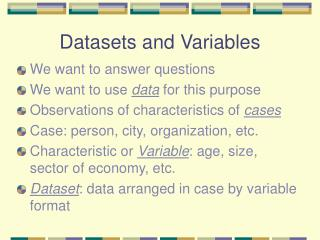Download PresentationDatasets and Variables

# Datasets and Variables - PowerPoint PPT PresentationDownload Presentation## Datasets and Variables

- - - - - - - - - - - - - - - - - - - - - - - - - - - E N D - - - - - - - - - - - - - - - - - - - - - - - - - - -
##### Presentation Transcript

1. Datasets and Variables • We want to answer questions • We want to use data for this purpose • Observations of characteristics of cases • Case: person, city, organization, etc. • Characteristic or Variable: age, size, sector of economy, etc. • Dataset: data arranged in case by variable format

2. Datasets • Cases • Variables

3. Variables • Measures or observations of a case’s • traits • characteristics • qualities • attributes • amounts • quantities • etc.

4. Errors in variables • Missing values • Measurement errors • mistakes in • Reporting • Remembering • Recording • Lies, etc. • Quality of answer ~ quality of data

5. Types of variables • Categorical: nominal (name) • Ordinal: (name and order) • Measurement: interval and ratio • Interval (name, order, and unit of measure) • Ratio (name, order, unit, and true zero point)

6. Summarizing Data • Frequency Distributions • For measurement variables • For categorical variables

7. Frequency Distribution • For Categorical variables (table) Variable Value Frequency Proportion (Percent) Dem 17 .425 42.5% Rep 7 .175 17.5 Ind 16 .400 40.0 n=40 1.000 100.0% f f/n f/n * 100

8. Frequency Distribution • For Measurement variables (table) Variable Value Frequency Proportion (Percent) 0 19 .44 44 1 10 .23 23 … 7 2 .05 5 n=43 1.00 100

9. Frequency Distribution • For Categorical variable (bar chart)

10. Frequency Distribution • For Measurement variable (histogram)

11. Frequency Distribution • Frequency, f - count the number of cases that have the same value of a variable • Total cases, n - count all the cases • Proportion, p = f/n • Percentage, % = 100 * p

12. Dataset • Individual cases • e.g. stats.dta dataset: characteristics of individuals: age, msat, gender • Aggregate data (groups of individual cases) • e.g. college1.dta dataset: characteristics of individuals: age, msat, gender averaged for groupings of students by college

13. Populations and Samples • Population: all the relevant cases. The entire set. • Sample: some portion of the population • haphazard (e.g., whomever you meet) • systematic (e.g., every 10th person) • representative (all possibilities included) • random (every case in the population has a fixed probability of being included in the sample, often equal probability)

14. Populations • Best information • Expensive or impossible to observe

15. Samples • Easier to get • Less expensive • Less accurate - but, can be very accurate depending upon type of sample

16. Hints for good grade • Do all assigned exercises and turn them in on time • Do all other exercises for yourself to be sure you understand • Read and study the text, before and after lectures -- several times • Statistics is a language -- learn (memorize) the vocabulary and concepts